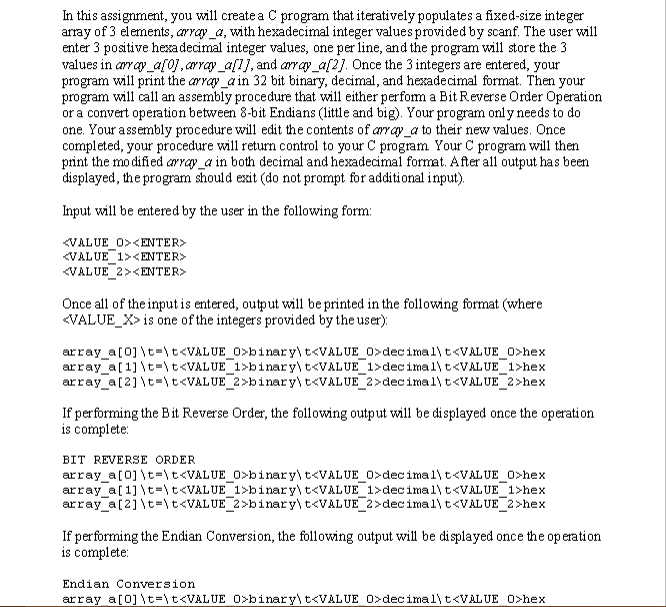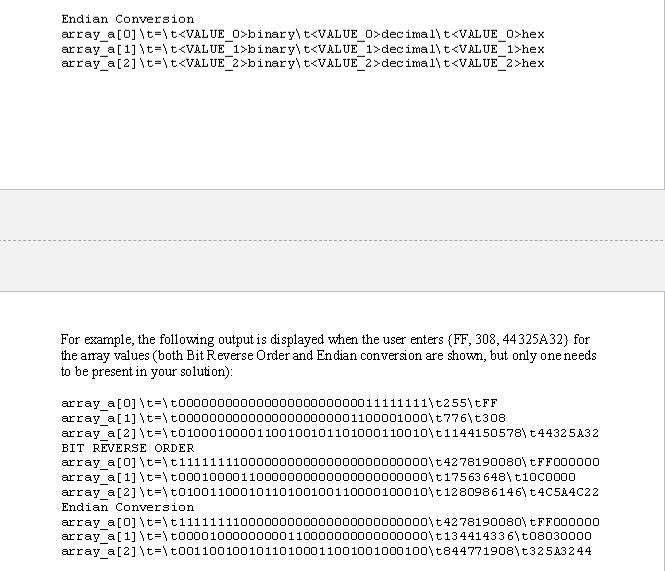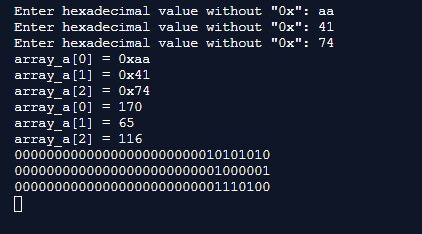Homework Help Question & Answers

JUST GIVE ME THE C CODE FOR THIS QUESTION. I GOT THE ASSEMBLY CODE.JUST GIVE ME THE C CODE FOR THIS QUESTION. I GOT THE ASSEMBLY CODE.

Endian Conversion ar raya [ 2] \t=\ t«VALUE 2 >binary| t«VALUE 2 >decimal丶t«VALUE 22hex - - 一 For example, the following output is displayed when the user enters (FF, 308, 44325A32) for the array values (both Bit Reverse Order and Endian conversion are shown, but only oneneeds to be present in your solution) ar raya [O] \t=\t000000000000000000000000 11111111\t255\tFF array a\t-\ t00000000000000000000001100001000t7761t308 ar ray a\t=\t01000100 0 0 1 100 100101101000110010\t1144150578\t44325A32 BIT REVERSE ORDER ar ray a\t=\ t 1 1111111000000000000000000000000丶t4278190080\tFF00 0000 array a\t-\t00010000110000000000000000000000 t 17563 648 t10co000 ar ray a\t=\t0 1001100010110100100110000100010\t12809 8 6 14 6\t4C5A4C22 Endian Conversion ar ray a\t=\ t 1 1111111000000000000000000000000丶t4278190080\tFF00 0000 ar ray a\t=\t00001000 000000110000000000000000\t134414336\t08030000 array_a t-t001100100101101000110010010001001t844771908 t325A3244

#include <stdio.h>

int main(void)
{
unsigned int array_a,a;
int d,c,k,i;
//%x for hex input
printf("Enter hexadecimal value without \"0x\": ");
scanf("%x", &array_a);
printf("Enter hexadecimal value without \"0x\": ");
scanf("%x", &array_a);
printf("Enter hexadecimal value without \"0x\": ");
scanf("%x", &array_a);
//hex
printf("array_a = 0x%x \narray_a = 0x%x \narray_a = 0x%x \n", array_a,array_a,array_a);
//decimal
printf("array_a = %d \narray_a = %d \narray_a = %d \n", array_a,array_a,array_a);

for(i=0;i<3;i++){
//pick one element at a time to convert
a = array_a[i];
//convert decimal to binary
//c starts from 31 as we are doing 32 bit representation
for (c = 31; c >= 0; c--)
{
k = a >> c;
//k is and with 1 if it is 1 output will be 1 0 otherwise
if (k & 1)
printf("1");
else
printf("0");
}

printf("\n");
}

return 0;
}

outputAdd Answer of: JUST GIVE ME THE C CODE FOR THIS QUESTION. I GOT THE ASSEMBLY CODE.
More Homework Help Questions Additional questions in this topic.

• 1) y/z for y =-6 z = -20 i got: -6/-20 = 0.3 2) 2y/-z y= -5 z = -12 i got: 2(-5)/-12 = -10/-12 = 0.83 3) y + z/2z y= -4 z = 8 i got: -4 +8 / 2(8) = 4/16 = 1/4 4) -2y + 1/-10 y= 3 z = 10 i got: -2(3) + 1/-10 = -5/-10 = 0.5 are these corr

Need Online Homework Help?

Get FREE EXPERT Answers
WITHIN MINUTES
Related Questions